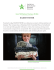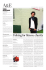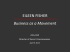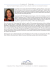# Document 6517344

## Transcription

Document 6517344
```c Korbinian Strimmer - Statistical Thinking - Draft June 13, 2013
4
What is information?
We describe various types of information inherent in probabilistic models, many
of which play an important role in statistical inference.
4–1 Overview
There are several notions of information related to random variables. While
quite different all of them are closely related (Figure 4–1).
The most fundamental and most important is the Kullback-Leibler (KL) information, or equivalently, the Boltzmann entropy. It measures the similarity of
two probabilistic models. The Shannon entropy and Fisher’s information matrix
are further information quantities, both of which can be directly derived from
Kullback-Leibler information. We also discuss mutual information as an important application of Kullback-Leibler information to quantify independence.
Figure 4–1: Measures of information discussed in this chapter, and their relationships.
1
c Korbinian Strimmer - Statistical Thinking - Draft June 13, 2013
4–2 Kullback-Leibler information
The Kullback-Leibler (KL) information is a fundamental quantity in probability
and statistics that measures the similarity of two distributions. It is hard to
overstate the importance of KL information: many statistical procedures for inference use KL information either directly or undirectly, including the likelihood
and penalized likelihood approaches. KL information was formally introduced
in the 1950s but the same quantity was already discovered almost a century
earlier in the 1870s by the Ludwig Boltzmann, a physicist famous for developing
the stochastic underpinnings of thermodynamics.
Suppose we have two models defined on the same sample space, one with with
distribution F and a second one with distribution G. Then the expectation
f (X)
Equation 4–1
I KL ( F; G ) := EF log
g( X )
is the Kullback-Leibler information of G with regard to F. Applying Equation 2–2 with h( X ) = log{ f ( X )/g( X )} we get to the formulas

f ( xi )

d

for a discrete variable, and
∑i=1 f ( xi ) log g( xi )
I KL ( F; G ) = R

∞
f (x)

 −∞ f ( x ) log g( x) dx for a continous variable.
With a negative sign attached KL information becomes the Boltzmann entropy
B( F; G ) = − I KL ( F; G ) .
Mathematically, I KL belongs to the class of f -divergences, and a further common
name it is relative entropy.
The KL information has the following properties:
(i) It is always non-negative: I KL ( F; G ) ≥ 0.
(ii) I KL = 0 only if the two models F and G are identical.
(iii) The KL divergence is not symmetric: I KL ( F; G ) 6= I KL ( G; F ).
(iv) I KL is invariant against transformations of the sample space. If instead of
X the random variable Y = h( X ) is considered, where h is an invertible
function, and the distributions G and F are transformed accordingly (cf.
Equation 2–7), the Kullback-Leibler information remains the same. Thus,
I KL is a geometric quantity that is independent of the choice of a specific
coordinate system.
2
What is information?
c Korbinian Strimmer - Statistical Thinking - Draft June 13, 2013
The KL information is a measure of the difference between two distributions,
i.e. the larger I KL the further apart are the two models. However, because of
the lack of symmetry in the two arguments it is not a metric. Therefore, the
term KL distance must be avoided and the expression KL divergence should be
used instead. A symmetric version of I KL is given by the Jeffreys information
J ( F; G ) = I KL ( F; G ) + I KL ( G; F ).
The asymmetry in the arguments of I KL is not coincidental — the two models F
and G play very different roles. In particular, the expectation in Equation 4–1
is with respect only to F, and not to G. In typical application of I KL the model
F is considered to be the true model and G the approximative model. In this
setting the KL divergence measures, loosely speaking, the information lost by
using candidate model G rather than the correct model F. Note that the minus
sign in the definition of the Boltzmann entropy explicitly accounts for the fact
that there is always a loss when using a model different from the true model.
An alternative, probabilistic interpretation of the KL divergence is given in the
next section where Equation 4–1 is derived from a combinatorical point of view.
Example 4–1: KL divergence between two normal distributions
Consider two univariate normal models F0 and F with means µ0 and µ and variances σ02
and σ2 . After some calculation we get for the KL information
I KL ( F0 ; F ) =
1
2
2
σ0
(µ − µ0 )2 σ02
+
−
log
−
1
.
σ2
σ2
σ2
Equation 4–2
This expression is invariant against changes of scale and location — but not against other
more general coordinate transformations. This not in contradiction with the geometric
property of I KL because general changes of units will lead outside the normal family, and
thus the above formula is not appropriate.
For multivariate normal F0 and F of dimension d with means µ0 and µ and covariances
Σ0 and Σ the KL information is given by
1
KL
T −1
−1
−1
I ( F0 ; F ) =
(µ − µ0 ) Σ (µ − µ0 ) + tr Σ Σ0 − log det Σ Σ0 − d ,
2
which for d = 1 reduces to Equation 4–2. For identical covariances (Σ = Σ0 ) the KL
information becomes symmetric and proportional to (µ − µ0 ) T Σ−1 (µ − µ0 ), which is
known as the squared Mahalanobis distance.
Example 4–2: KL divergence between two exponential distributions
The KL divergence from an exponential distribution with mean µ0 to an approximating
exponential with mean µ has a similar form as in the normal example. With density
f ( x |µ) = µ1 e− x/µ we get
I KL ( F0 ; F ) =
µ0
µ
− log 0 − 1 .
µ
µ
4–2 Kullback-Leibler information
3
c Korbinian Strimmer - Statistical Thinking - Draft June 13, 2013
Example 4–3: Pearson’s chi-squared statistic as approximation to KL divergence
Applying a Taylor series expansion of p around q we can approximate
p log
p
( p − q )2
≈ ( p − q) +
.
q
2q
With this in mind, twice the KL divergence between two discrete distributions P and Q
with dimension d and probability masses pi and qi is
d
G2 = 2 I KL ( P; Q) = 2 ∑ pi log
i =1
pi
qi
d
≈
( p − q )2
= χ2 .
q
i =1
∑
Thus, Pearson’s chi-squared goodness of fit statistic between an observed P and an
expected Q may be viewed as approximation to (twice) the KL divergence between P and
Q. The quantity G2 = 2I KL ( P; Q) is also known as the G-squared statistic, or likelihood
ratio chi-squared statistic.
Example 4–4: Approximation of a gamma distribution
Figure 4–2: A gamma density and two approximating models (log-normal and normal).
We would like to approximate a gamma distribution with shape parameter α = 3 and
scale parameter β = 1 by either a log-normal distribution or a normal distribution with
matching mean (3) and variance (3) — see Figure 4–2. Which of the two candidates
approximates the gamma model best?
For the answer we compute the KL information between the gamma and the two approximating models by numerical integration and obtain I KL = 0.0594 for the matching
4
What is information?
c Korbinian Strimmer - Statistical Thinking - Draft June 13, 2013
log-normal and 0.1207 for the normal distribution. This confirms what is evident already
by visual inspection: the KL number to the log-normal model is smaller and thus it is a
better approximation of the gamma model than the normal distribution.
4–3 Boltzmann’s derivation and probabilistic interpretation
The definition of the Kullback-Leibler divergence in Equation 4–1) as an expectation of log-densities can be derived and better understood by a simple argument
which goes back Ludwig Boltzmann.
We consider a simple urn experiment where we randomly allocate n elements
to m different compartments (in the original setting these correspond to gas
particles and energy levels). The assignment of a single element to one of the urns
is governed by a given distribution G, with probability mass function gi = g( xi ).
The probability to see a specific allocation n1 , . . . , nm with n = ∑im=1 ni is given
by multinomial probability
Ψ = Pr(n1 , . . . , nm | g1 , . . . , gm ) =
n!
∏im=1 ni !
m
∏ gi i .
n
i =1
If we instead of probability look at log-probability
m
m
i =1
i =1
log Ψ = log(n!) − ∑ log(ni !) + ∑ ni log( gi ) .
For large n and ni the Sterling approximation log(n!) ≈ n log(n) − n simplifies
the terms containing factorial functions, so that we get
log Ψ
m
ni
+ ∑ ni log( gi )
=
− ∑ ni log
n
i =1
i =1
m
ni /n
ni
.
= −n ∑ log
n
gi
i =1
large sample
m
For large n the observed frequencies ni /n approach a probability mass function
f i = f ( xi ), so that finally we get the asymptotic identity
large sample
1
log Ψ
=
B( F; G ) = − I KL ( F; G ) .
n
Equation 4–3
In essence, the KL information is the negative log probability per data point to
observe the correct model F under the specified model G. This is really quite a
remarkable result, in particular as it exhibits a clear Bayesian flavor: whenever
we use I KL we implicitly evaluate the probability of the true model!
4–3 Boltzmann’s derivation and probabilistic interpretation
5
c Korbinian Strimmer - Statistical Thinking - Draft June 13, 2013
4–4 Expected Fisher information
The expected Fisher information matrix I Fisher , which plays a very important
role in likelihood and Bayes theory, is a local version of KL information.
Let’s consider a family of distributions Fθ indexed by the parameter vector
θ = (θ1 , . . . , θd ) T of dimension d. For a specific θ we compare the distribution
Fθ with its neighbor Fθ+ε obtained by shifting the parameter vector θ by some
small amount ε. The KL divergence between Fθ and Fθ+ε is
KL
I ( Fθ; Fθ+ε ) = EFθ log f ( X |θ) − log f ( X |θ + ε) .
By quadratic approximation of the last term in the brackets as log f ( X |θ + ε) ≈
log f ( X |θ) + ∇ log f ( X |θ) T ε + 12 ε T ∇ T ∇ log f ( X |θ)ε and taking into account
that the expectation of ∇ log f ( X |θ) vanishes (see Problems) we get
1
I KL ( Fθ; Fθ+ε ) ≈ EFθ − ε T ∇ T ∇ log f ( X |θ)ε
2
Equation 4–4
1 T Fisher
= ε I
(θ) ε
2
with the d × d matrix I Fisher (θ) given by
Fisher
T
I
(θ) = −EFθ ∇ ∇ log f ( X |θ) .
Equation 4–5
The expected Fisher information I Fisher (θ) is, unlike KL divergence, symmetric
and provides a local metric in the space of distributions indexed by the parameter
vector θ. In a later chapter we will dicuss further geometrical properties of the
Fisher information, e.g., it is formally a metric tensor describing the manifold of
models.
As a local version of KL information I Fisher is invariant against a change of
coordinates in the sample space. However, if the index θ is changed to φ(θ)
then expected Fisher information transforms according to
I Fisher
(φ) = J θ (φ)T I Fisher
(θ(φ)) J θ (φ) ,
φ
θ
Equation 4–6
where J θ (φ) is the Jacobi matrix containing the partial derivatives of θ(φ).
Example 4–5: Expected Fisher information of the normal model
We consider a normal distribution with mean µ and variance σ2 . The expected Fisher
information matrix may be directly computed via Equation 4–5 but this is a bit tedious.
A much faster route consists of reusing the result from Example 4–1. Using Equation 4–2
6
What is information?
c Korbinian Strimmer - Statistical Thinking - Draft June 13, 2013
we compute the KL divergence of N (µ, σ2 ) from N (µ + ε 1 , σ2 + ε 2 ). Approximating the
logarithm log(1 + x ) ≈ x − x2 /2 we obtain
I
KL
1
( N (µ + ε 1 , σ + ε 2 ); N (µ, σ )) ≈
2
2
2
ε21
ε22
+
σ2
2σ4
so that by comparison with Equation 4–4 we finally get
I
Fisher
2
(µ, σ ) =
1
σ2
0
0
!
.
1
2σ4
This result is quite interesting: for the location parameter µ we get I Fisher (µ) = σ12 , i.e.
the expected Fisher information is a constant independent of µ, and this constant equals
the inverse variance, i.e. the precision. In contrast, for the parameter σ2 the Fisher
information I Fisher (σ2 ) = 2σ1 4 does depend on the parameter σ2 .
Example 4–6: Expected Fisher information for the standard deviation
In the previous example, we computed the expected Fisher information of the variance,
I Fisher (σ2 ). What happens if we reparameterize and consider instead of σ2 the standard
deviation σ as our primary parameter ?
From Equation 4–2 we get I KL ( N (µ, (σ + ε)2 ); N (µ, σ2 )) ≈ 21 ε( σ22 )ε which implies
I Fisher (σ) = σ22 . Note that I Fisher (σ) differs from I Fisher (σ2 ) = 2σ1 4 .
The same result can also be directly obtained by applying the transformation
rule of
1
0
Equation 4–6. With θ = (µ, σ2 ) T , φ = (µ, σ ) T , and J θ (φ) =
we get
0 2σ
I Fisher (µ, σ) =
1
σ2
0
0
2
σ2
!
.
This result generalizes to arbitrary location-scale families (see Problems): a location
parameter always has constant Fisher information, and for any scale parameter σ the
Fisher information is proportional to 1/σ2 .
Example 4–7: Expected Fisher information in a collection of iid random variables
If we consider a set of n independent and identically distributed (iid) random variables
X = ( X1 , . . . , Xn ) what is the total expected Fisher information in X?
For independent random variables the joint density f ( x|θ) = f ( xi |θ)n is the product
of the individual densities. Plugged into Equation 4–5 this results in nI Fisher (θ) where
I Fisher (θ) is the information for θ in any of the components Xi . Thus, the total Fisher
information is the sum of the Fisher information in each individual variable.
4–4 Expected Fisher information
7
c Korbinian Strimmer - Statistical Thinking - Draft June 13, 2013
4–5 Mutual information
A further important and very useful quantity derived from KL information is
the mutual information between two random variables.
Mutual information is a measure of statistical dependencies. Two variables X1
and X2 are said to be stochastically independent if the joint density f ( x1 , x2 )
factorizes into the product of the marginal densities g1 ( x1 ) and g2 ( x2 ). The
mutual information is the KL information from the joint density to the product
density,
MI( X1 , X2 ) =
Z ∞ Z ∞
−∞ −∞
f ( x1 , x2 )
f ( x1 , x2 ) log
g1 ( x 1 ) g2 ( x 2 )
dx1 dx2 .
Equation 4–7
Mutual information inherits all favorable properties of KL information including
transformation invariance. It is applicable to both discrete and continuous variables. Furthermore, by construction mutual information is symmetric, always
non-negative and becomes zero only if the variables are independent.
Example 4–8: Mutual information between two normal distributed variables
Consider two variables X1 and X2 following a joint normal distribution with means µ1
and µ2 , variances σ12 and σ22 and correlation ρ. A bit of calculation shows that the mutual
information between X1 and X2 equals
1
MI( X1 , X2 ) = − log(1 − ρ2 ) .
2
Hence, for normal variables mutual information is a function of the correlation. If the
correlation vanishes (ρ = 0) then we have MI = 0. Conversely, for perfect correlation
(ρ = 1) the mutual information will be infinite. Intuitively, this makes perfect sense, as
correlation is a measure of how much information is contained in one random variable
for the other. However, it is also important to keep in mind that the normal model is very
special — for non-normal random variables zero correlation does in general not imply
Example 4–9: Mutual information between two categorical variables
The mutual information between two discrete variables X1 and X2 with dimensions n1
(1)
and n2 is straightforward to compute. If the marginal mass functions are denoted by πi
(2)
and π j with i = 1, . . . , n1 and j = 1, . . . , n2 , and the joint probability mass function is
given by the n1 × n2 table πij the mutual information is
MI( X1 , X2 ) = Σin=1 1 Σnj=2 1 πij log
8
What is information?
πij
(1) (2)
πi π j
c Korbinian Strimmer - Statistical Thinking - Draft June 13, 2013
which can be approximated using a Taylor series (see Example 4–3) as
(1) (2) 2
1 n1 n2 πij − πi π j
1
MI( X1 , X2 ) ≈ Σi=1 Σ j=1
= χ2 ,
(1) (2)
2
2
π π
i
j
yielding (half) the Pearson’s chi-squared statistic of independence.
4–6 Shannon entropy
Probably the most widely known information criterion is the Shannon entropy.
For a discrete random variable with distribution F the Shannon entropy is
defined by
m
H ( F ) = − ∑ f ( xi ) log f ( xi ) .
Equation 4–8
i =1
This expression looks very similar to the Boltzmann entropy B( F, G ), and indeed
for G uniform with g( xi ) = 1/m there is the relationship
H ( F ) = B( F, G ) + log m .
Therefore, the Shannon entropy simply measures the difference of the uniform
distribution and the distribution of the random variable of interest. The Shannon
entropy H ( F ) is always greater than or equal to zero, and it reaches its maximum
value (log m) if F is itself the uniform distribution.
Shannon entropy also has a close link to mutual information. For discrete
variables mutual information can be written in terms of the Shannon entropies
of the marginal and joint distributions,
MI( X1 , X2 ) = H ( X1 ) + H ( X2 ) − H ( X1 , X2 ).
Thus, mutual information is the difference of the joint entropy and the sum of
the two marginal entropies.
Surprisingly, it is rather difficult to generalize Shannon entropy from a discrete
to a continuous random variable. Replacing the summation in Equation 4–8 by
an integral leads to differential entropy
H ( F) = −
Z ∞
−∞
f ( x ) log f ( x )dx .
Unfortunately, differential entropy is not a fully satisfying extension of Shannon
entropy, as it exhibits a number of unfavorable defects. For instance, it can take
on negative as well as positive values. Moreover, differential entropy is not
invariant under coordinate transformations, so unlike the KL information its
value is dependent on the choice of units.
4–6 Shannon entropy
9
c Korbinian Strimmer - Statistical Thinking - Draft June 13, 2013
These issues can be traced back to the divergence of the above term log m in the
process of going from a discrete to continuous variable, or equivalently, to the
problem of generalizing the discrete uniform distribution G to the continuous
case. Hence, we recommend using Shannon entropy only in its original discrete
domain, and otherwise to employ KL information.
Example 4–10: Negative differential entropy
A simple example to demonstrate that differential entropy behaves differently than the
Shannon entropy is the uniform
distribution U (0, a). Its density within its support is
Ra
f ( x ) = 1a so that H = − 0 1a log( 1a ) dx = log a. Thus, for 0 < a < 1 the differential
entropy of U (0, a) is negative.
4–7 Bibliographic notes
Shannon entropy is introduced in Shannon (1948) and Kullback-Leibler information in Kullback and Leibler (1951). Akaike (1985) traces the origins of KL
information back to Boltzmann’s entropy and also describes its probabilistic
interpretation. In Jaynes (2003), section 11.4, a similar derivation is given for the
Shannon entropy. Jaynes also discusses the problem of generalizing Shannon
entropy to the continuous case (section 12.3). Fisher information goes back to
Fisher (1925) and its relationship to KL information is given in Kullback and
Leibler (1951). The fact that expected Fisher information is a metric tensor was
first noted by Rao (1945).
4–8 Problems
4–1 Show that KL information is either positive or zero. Hint: use Jensen’s
inequality (Equation 2–8).
4–2 Show that KL information and Fisher information is invariant against
coordinate transformations in the sample space.
4–3 Verify Example 4–1 and Example 4–4.
4–4 Write a computer program demonstrating that the Boltzmann derivation
of KL information is correct.
4–5 Let S1 = ∇ log f ( X |θ). Show that E(S1 ) = 0 and Var(S1 ) = I Fisher (θ).
Hint: use the fact that f ( x |θ) is a probabilty density and that expectation
and differentiation can be exchanged.
4–6 Show that the expected Fisher information I Fisher (θ ) is constant if θ is a
location parameter, and that it is proportional to 1/θ 2 for a scale parameter.
10
What is information?
c Korbinian Strimmer - Statistical Thinking - Draft June 13, 2013
4–7 Compute the expected Fisher information for the parameters of the Bernoulli,
Binomial, Poisson, and Cauchy distributions.
'
\$
In a nutshell
• There are many different concepts of information. They are all
related, concern the relationships between two distributions, and
quantify the information contained in random variable for another.
• The most fundamental quantity is Kullback-Leiber information, or
equivalently, Boltzmann entropy. It has many favorable properties,
including transformation invariance.
• The Boltzmann entropy has probabilistic interpretation as the logprobability to observe the true model under a specified generating
model.
• Pearson’s chi-squared statistic is an approximation to (twice) the
KL divergence.
• Expected Fisher information is a local version of KL information
and has a geometrical meaning as a metric in the space of models.
• Mutual information measures independence and is an important
application of KL information.
• Shannon entropy can be viewed as a special case of KL information
where the approximating model is the uniform distribution. It is
problematic to apply for continuous random variables.
Related chapters
&
Likelihood, AIC, Geometry
%
4–8 Problems
11
```

### Fisher Vineyards ~ Featuring Cameron Fisher! TUESDAY, JUNE 9th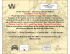### Fisher Cat Martes Pennanti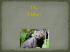### Former`Lolita`Amy Fisher sets Long Island on its ear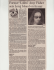### 3rd CEE Cyber and Information Security Showcase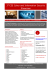### The Jonathon Lipnicki Theory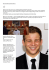### Fisher Parent News-November 2015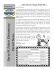### eileen fisher - American Sustainable Business Council